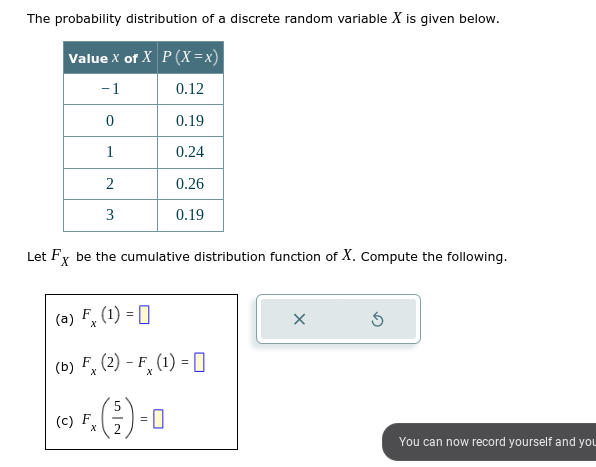Home / Expert Answers / Statistics and Probability / the-probability-distribution-of-a-discrete-random-variable-x-is-given-below-let-f-x-pa746

# (Solved): The probability distribution of a discrete random variable $$X$$ is given below. Let $$F_{X}$$ ...The probability distribution of a discrete random variable $$X$$ is given below. Let $$F_{X}$$ be the cumulative distribution function of $$X$$. Compute the following. (a) $$F_{x}(1)=$$ (b) $$F_{x}(2)-F_{x}(1)=$$ (c) $$F_{x}\left(\frac{5}{2}\right)=$$

We have an Answer from Expert